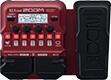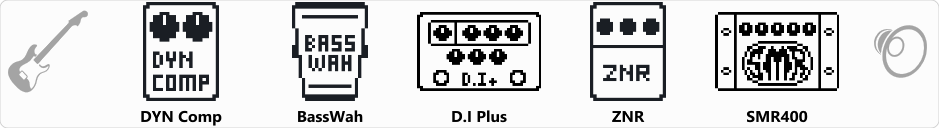# B1X Alex

Discussion in 'Zoom B1/B1X Four' started by Isellgood, Mar 4, 2023.

1. B1X AlexDevice: Zoom B1X Four
Firmware: 2.00

Name on device: WahDist
Optimized for: Guitar Amp

Effects chain:This distorted wah sound, which combines BassWah and D.I Plus, can be used for both solo and backing parts.

Effect: "DYN Comp" (Dynamics), active - "yes"
"Sense" = 8
"Attack" = Fast
"Tone" = 66
"Volume" = 50

Effect: "BassWah" (Pedal), active - "yes"
"Frequency" = 77
"Range" = 50
"Dry" = 20
"Volume" = 80

Effect: "D.I Plus" (Overdrive / Distortion), active - "yes"
"Bass" = 50
"MID" = 71
"Treble" = 37
"Color" = On
"CHAN" = DIST
"Blend" = 70
"Gain" = 80
"VOL" = 65

Effect: "ZNR" (Dynamics), active - "yes"
"Detect" = GTRIN
"Depth" = 100
"Threshold" = 35
"Decay" = 0

Effect: "SMR400" (Amp simulator), active - "yes"
"Bass" = 7.5
"MID-F" = 1.0kHz
"Middle" = 6.0
"Treble" = 0.0
"Gain" = 50
"ENHNC" = 0
"Volume" = 55

Patch Volume: 44